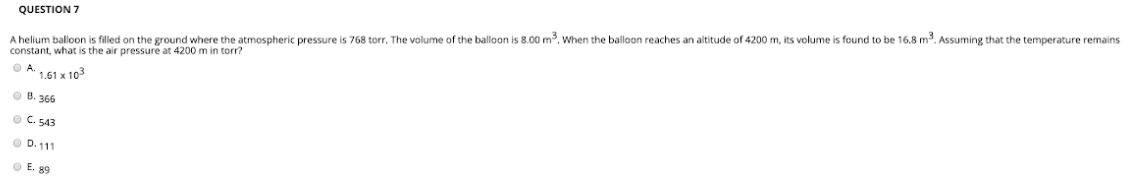# A helium balloon is filled on the ground where the atmospheric pressure is 768 torr. The volume of the balloon is 8.00 m^3. When the balloon reaches an altitude of 4200 m, its volume is found to be 16.8 m^3. Assume that the temperature remains constant, what is the air pressure at 4200 m in torr? A. 1.61 x 10^3 B. 366 C. 543 D. 111 E. 89